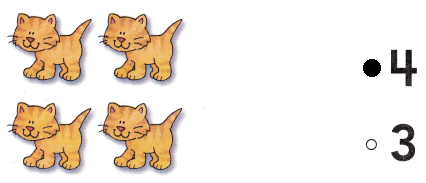# Texas Go Math Kindergarten Lesson 1.3 Answer Key Model Count, and Write 3 and 4

Refer to our Texas Go Math Kindergarten Answer Key Pdf to score good marks in the exams. Test yourself by practicing the problems from Texas Go Math Kindergarten Lesson 1.3 Answer Key Model Count, and Write 3 and 4.

## Texas Go Math Kindergarten Lesson 1.3 Answer Key Model Count, and Write 3 and 4

Explore

DIRECTIONS: Place a cube on each object in the set as you count them, Move the cubes to the five frame. Draw the cubes.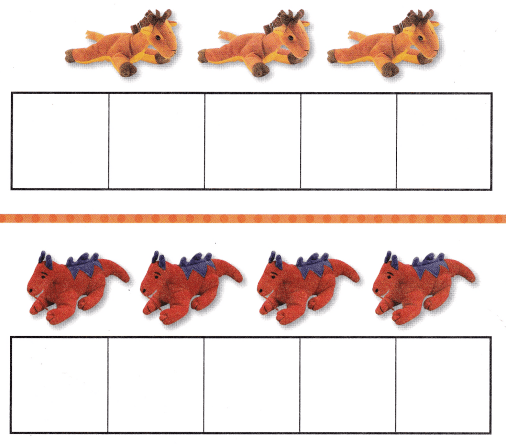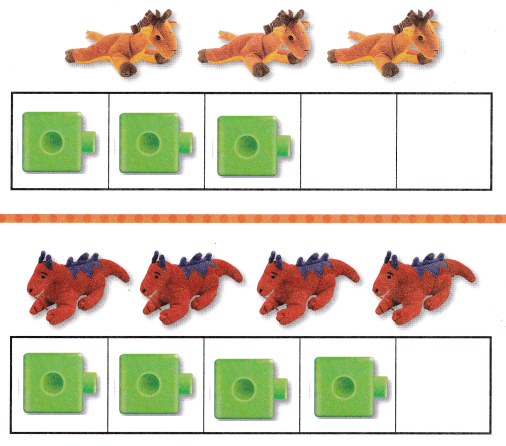Explanation:
Placed a cube on each object in the set as we count them,
Move the cubes to the five frame. Drawn the cubes.

Share and Show

DIRECTIONS: 1-2. Count the cubes. Say the number. Practice writing the number. 3-4. Count and tell how many. Write the number.

Question 1.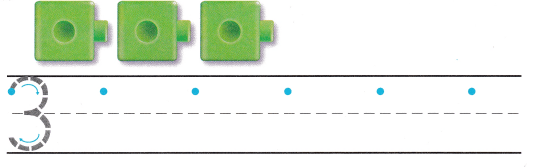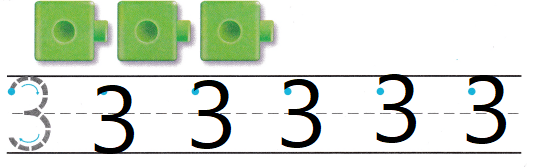Explanation:
Traced and written the number 3

Question 2.Explanation:
Traced and written the number 4

Question 3.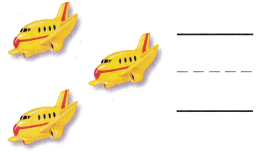Explanation:
Counted and written the number 3

Question 4.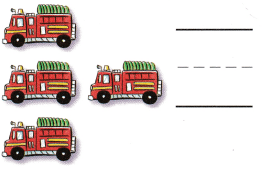Explanation:
Counted and written the number 4

DIRECTIONS: 5-10. Count and tell how many. Write the number.

Question 5.Explanation:
Counted and written the number 3

Question 6.Explanation:
Counted and written the number 4

Question 7.Explanation:
Counted and written the number 4

Question 8.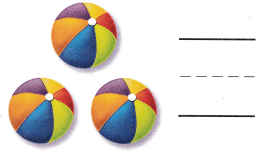Explanation:
Counted and written the number 3

Question 9.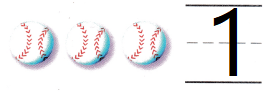Explanation:
Counted and written the number 3

Question 10.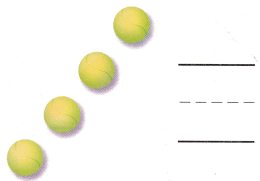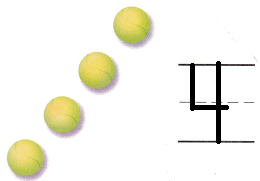Explanation:
Counted and written the number 4

HOME ACTIVITY • Ask your child to show o set of three or four objects. Have him or her write the number on paper to show how many objects.Explanation:
Written the number according to the objects shown by kid

DIRECTIONS: 11. Count the objects in each set. Circle the set of three objects. 12. Choose the correct answer. What number does the model show?

Problem Solving

Question 11.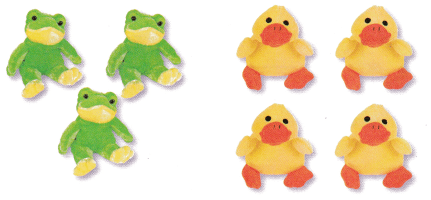Explanation:
Counted and written the number 3
And circled it

Question 12.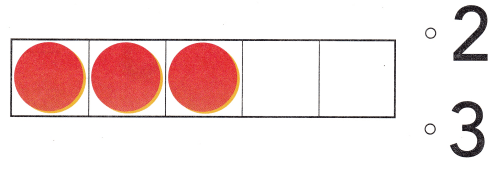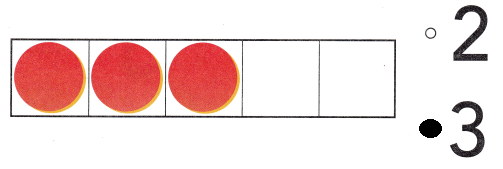Explanation:
Counted and bubbled the number 3

### Texas Go Math Kindergarten Lesson 1.3 Homework and Practice Answer Key

DIRECTIONS: 1-5. Count and tell how many. Write the number.

Question 1.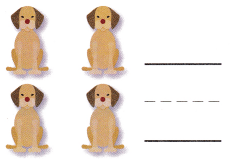Explanation:
Counted and written the number 4

Question 2.Explanation:
Counted and written the number 3

Question 3.Explanation:
Counted and written the number 3

Question 4.Explanation:
Counted and written the number 4

Question 5.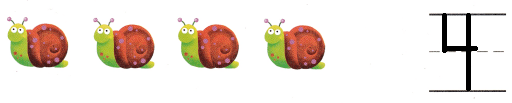Explanation:
Counted and written the number 4

DIRECTIONS: Choose the correct answer. 6. What number does the model show? 7. How many squirrels are there? 8. How many kittens are there?

Question 6.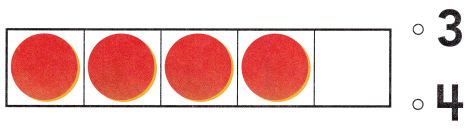Explanation:
Bubbled the number 4

Question 7.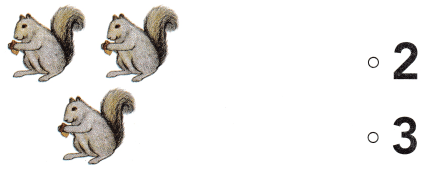Explanation:
Bubbled the number 3
There are 3 squirrels

Question 8.